# Resistance Formula

Resistance Formula

Electrical resistance is a property of materials that allow electric current to flow. Resistance opposes the flow of current. The unit of resistance is Ohms, which is represented with the Greek uppercase letter omega: Ω. Resistors are components of electric circuits. The resistance depends on the voltage across the resistor, and the current flowing through it.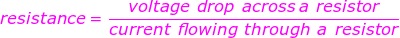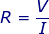R = resistance (Ohms, Ω)

V = voltage difference between the two ends of a resistor (Volts, V)

I = the current flowing through a resistor (Amperes, A)

Resistance Formula Questions:

1) In an electric circuit, a current of 3.00 A is flowing through a resistor. The voltage drop from one end of the resistor to the other is 120 V. What is the value of the resistance?

The resistance can be found using the formula: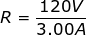R = 40.0 Ω

The resistance of the resistor in the circuit is 40.0 Ω.

2) A certain resistor in a circuit has a resistance of 300.0 . If the voltage difference across this resistor is 1500 V, how much electric current is flowing through the resistor?

The resistance is given in units of , meaning kilo-Ohms. One kilo-Ohm is equal to one thousand Ohms: 1 = 1000 Ω. The resistance is therefore: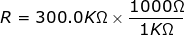R = 300000 Ω The current can be found by rearranging the formula: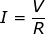I = 0.0050 A

The current flowing through the resistor is 0.0050 A. This can also be stated in milli-Amperes. One milli-Ampere is equal to one one-thousanth of an Ampere: 1 mA = 1/1000 A = 0.001 A. In these units, the current is: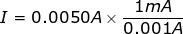I = 5.0 mA The current flowing through the resistor is 0.0050 A, which is equal to 5.0 mA.